Excel Round Off Formula can be used to remove decimal points from the data.

Apart from making the data presentable, it also makes the numbers easier to read and understand at first glance. Excel offers 4 different variations of the ROUND Formula:

Let’s look at each of these in-depth!

### ROUND Function

What does it do?

Rounds a number to the nearest decimal based on your specified number of digits

Formula breakdown:

=ROUND(number, num_digits)

What it means:

=ROUND(the number, number of decimal places to round off)

Ever had the need to round off numbers?

I do it all the time in my financial calculations. For example, if I need to calculate percentage discounts and it gives me a number such as \$47.4189349, rounding it off to \$47.40 (round off to 1 decimal place) makes it so much more presentable!

In Excel, it’s very easy to do that with the ROUND formula!Watch How to Round off in Excel on YouTube and give it a thumbs-up!#### Follow the step-by-step tutorial on Excel Round off Formula and download this Excel workbook to practice along:

STEP 1: We need to enter the ROUND function:

### =ROUND(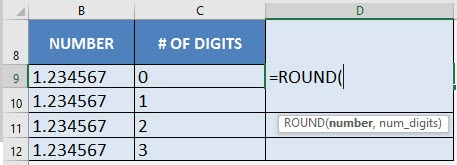STEP 2: The ROUND arguments:

### number

What is the number we want to round off?

Reference the cell that contains the number:

### =ROUND(B9,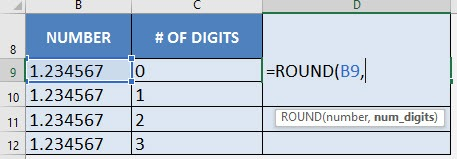### num_digits

Round off to how many digits?

Reference the cell that contains the number of digits:

### =ROUND(B9, C9)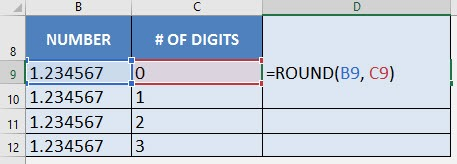STEP 3: Do the same for the rest of the cells by dragging the ROUND formula all the way down using the left mouse button.

Now you are able to Excel round off formula with a different number of places!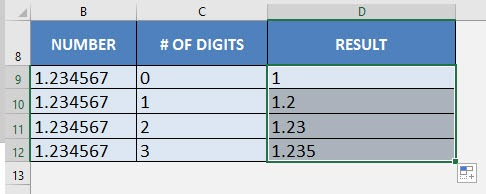Note: A negative sign before the rounding digit indicates how many places to the left of the decimal point should the number be rounded.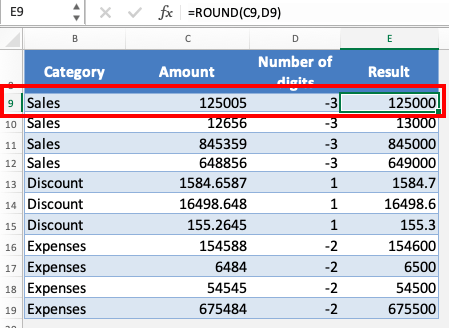### ROUNDUP Function

ROUNDUP Function is used to round the number up to the number of decimal points written.

For the ROUND function, if the number is less than 5 it is rounded down but for ROUNDUP it will always round up the numbers.

For Example –

### =ROUNDUP(104.1,0)

If you want to round up 104.1 to zero decimal places, ROUNDUP will provide the result as 105!

If the argument for the number of decimal points is

• Positive numbers – Excel will round to the right of the decimal point
• Negative numbers – Excel will round to the left of the decimal point
• Zero – Excel will round to the nearest 1.

We will show how to Excel round off formula using ROUNDUP below:

STEP 1: Enter the roundup formula

### =ROUNDUP(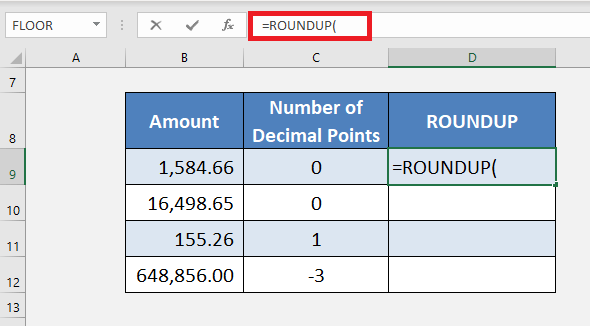STEP 2: Select the cell whose amount needs to be rounded

### =ROUNDUP(B9,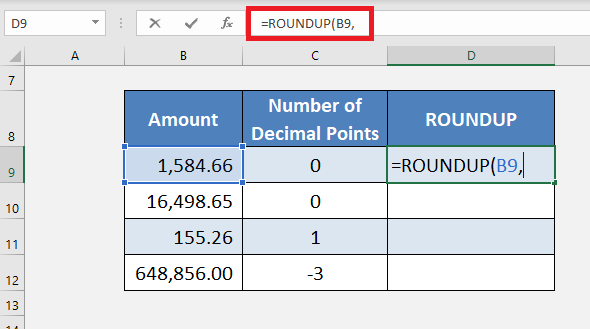STEP 3: Select the cell that contains the digits up to which the number needs to be rounded.

### =ROUNDUP(B9,C9)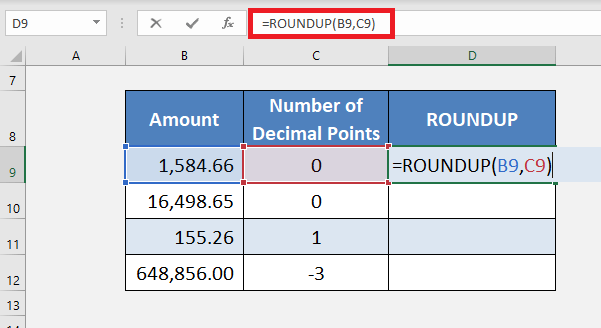The number of digits that the number needs to be rounded up to is entered here. It can be entered in a cell and the cell can be selected, or the digit can directly be written in the formula.

STEP 4: Copy-Paste the formula down to the remaining cells.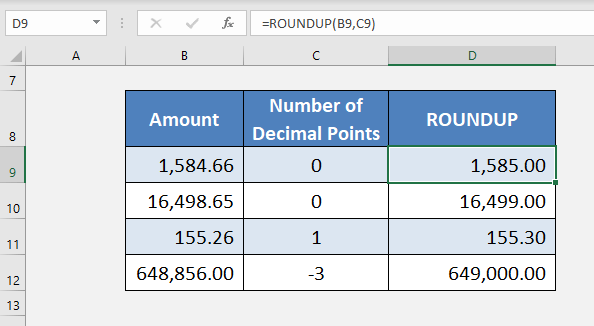As the formula is entered, the result gives the amount rounded up to the given decimal points

### ROUNDDOWN Function

Another variation is ROUNDDOWN that rounds down the number to the desired number of decimal places.

Here, the steps are the same except for selecting the ROUNDDOWN function instead of the ROUNDUP function.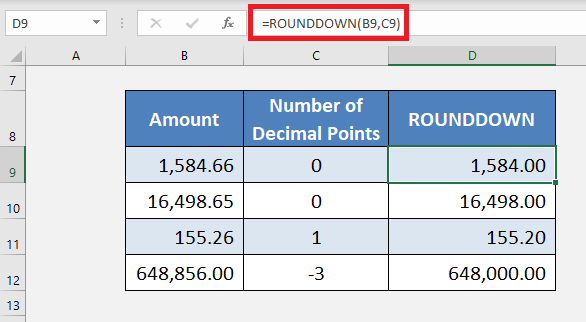For the amount 1584.66, the ROUNDUP formula provided the result as 1585 but ROUNDDOWN has provided the result as 1584!

### MROUND Function

It rounds the number to the nearest multiple of a number given. It will round the number up or down depending on the nearest multiple provided in the formula.

For example, this function can be used to;

• Round a price to the nearest multiple of 3
• Round a price to the nearest 0.05
• Round a time to the nearest 30 minutes.

Let us take an example and Excel round off formula to the nearest multiple:

STEP 1: Enter the MROUND formula

### =MROUND(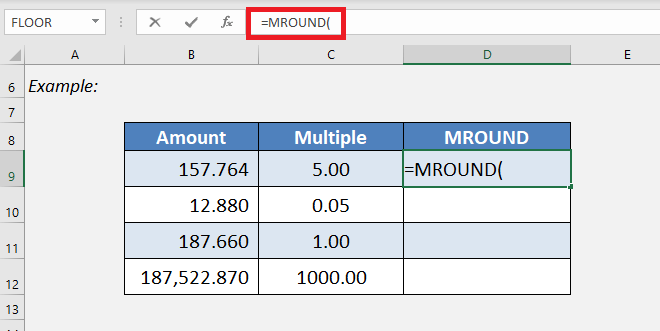STEP 2: Enter the first argument i.e. the number that should be rounded.

### =MROUND(B9,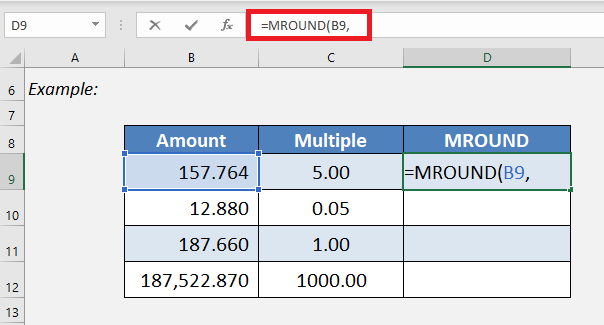STEP 3: Enter the second argument i.e. the multiple that the provided number should be rounded to.

### =MROUND(B9,C9)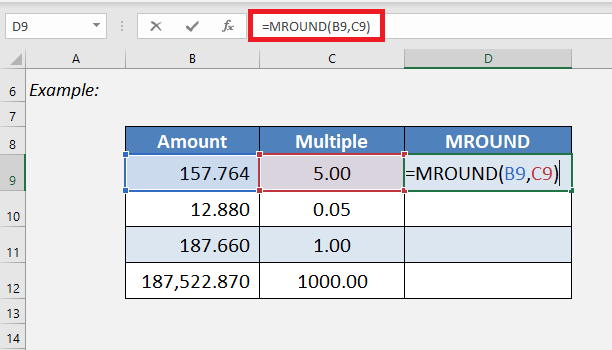Excel will round the number 157.764 to the nearest multiple of 5 i.e. 160!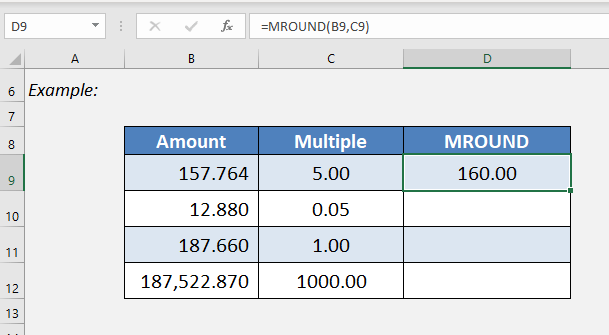STEP 4: Copy-paste the formula below.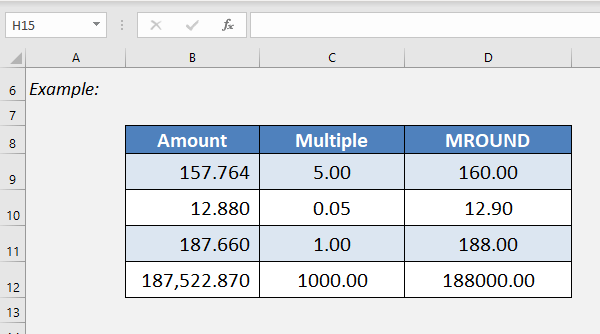This completes our tutorial on how to round off numbers in Excel!

### Conclusion

There are numerous ways to Round off formula in Excel and present the value in the best way possible. So whatever your requirements, Excel has a way.

Be sure to understand the difference and choose the right one to prevent unexpected results.